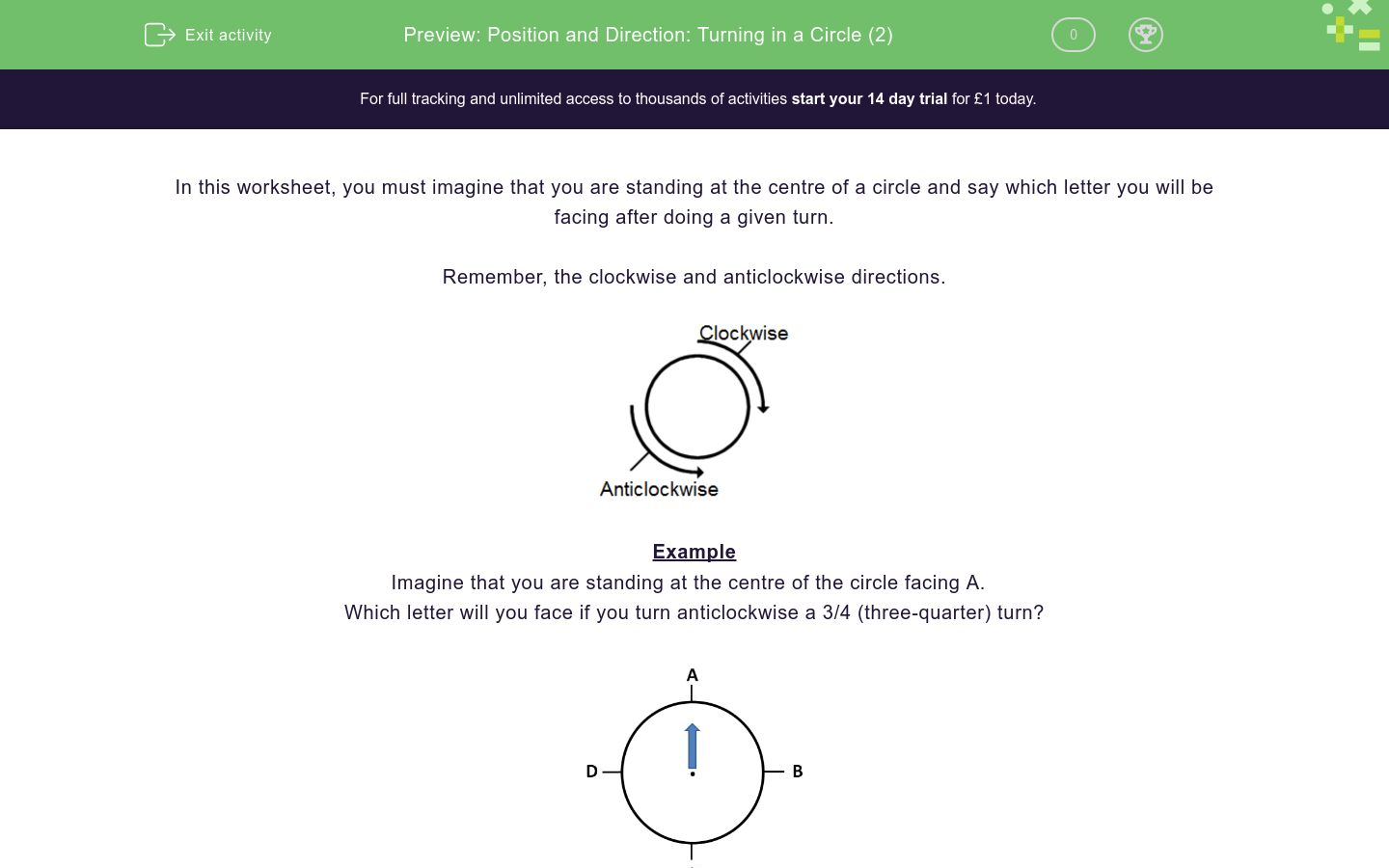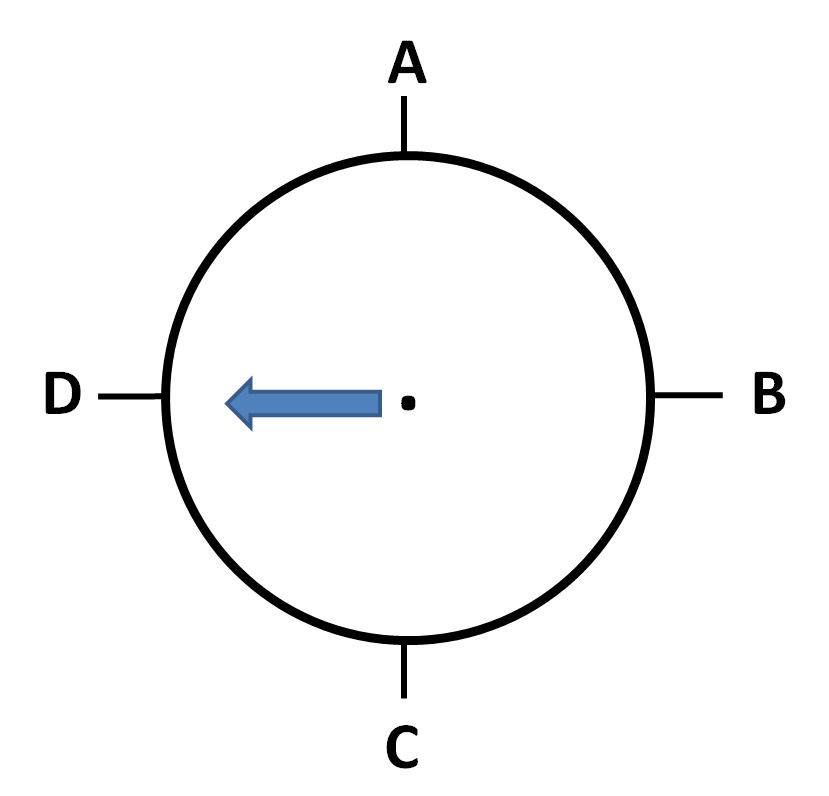# Position and Direction: Turning in a Circle (2)

In this worksheet, students give the result of quarter and half turns, clockwise.Key stage:  KS 1

Curriculum topic:   Geometry: Position and Direction

Curriculum subtopic:   Use Language of Position/Direction

Difficulty level:### QUESTION 1 of 10

In this worksheet, you must imagine that you are standing at the centre of a circle and say which letter you will be facing after doing a given turn.

Remember, the clockwise and anticlockwise directions.Example

Imagine that you are standing at the centre of the circle facing A.

Which letter will you face if you turn anticlockwise a 3/4 (three-quarter) turn?Imagine that you are standing at the centre of this circle facing A.

Say which letter you will be facing after doing the given turn.

A quarter turn anticlockwise.A

B

C

D

Imagine that you are standing at the centre of this circle facing B.

Say which letter you will be facing after doing the given turn.

A three-quarter turn clockwise.A

B

C

D

Imagine that you are standing at the centre of this circle facing B.

Say which letter you will be facing after doing the given turn.

A quarter turn clockwise.A

B

C

D

Imagine that you are standing at the centre of this circle facing A.

Say which letter you will be facing after doing the given turn.

A one and a half turn.A

B

C

D

Imagine that you are standing at the centre of this circle facing C.

Say which letter you will be facing after doing the given turn.

A one-and-a-half turn.A

B

C

D

Imagine that you are standing at the centre of this circle facing C.

Say which letter you will be facing after doing the given turn.

A three-quarter turn anticlockwise.A

B

C

D

Imagine that you are standing at the centre of this circle facing D.

Say which letter you will be facing after doing the given turn.

A three-quarter (3/4) turn clockwise.A

B

C

D

Imagine that you are standing at the centre of this circle facing A.

Say which letter you will be facing after doing the given turn.

A three-quarter (3/4) turn clockwise.A

B

C

D

Imagine that you are standing at the centre of this circle facing A.

Say which letter you will be facing after doing the given turn.

A three-quarter (3/4) turn anti-clockwise.A

B

C

D

Imagine that you are standing at the centre of this circle facing A.

Say which letter you will be facing after doing the given turn.

A two-and-three-quarter turn clockwise.A

B

C

D

• Question 1

Imagine that you are standing at the centre of this circle facing A.

Say which letter you will be facing after doing the given turn.

A quarter turn anticlockwise.D
• Question 2

Imagine that you are standing at the centre of this circle facing B.

Say which letter you will be facing after doing the given turn.

A three-quarter turn clockwise.A
• Question 3

Imagine that you are standing at the centre of this circle facing B.

Say which letter you will be facing after doing the given turn.

A quarter turn clockwise.C
• Question 4

Imagine that you are standing at the centre of this circle facing A.

Say which letter you will be facing after doing the given turn.

A one and a half turn.C
• Question 5

Imagine that you are standing at the centre of this circle facing C.

Say which letter you will be facing after doing the given turn.

A one-and-a-half turn.A
• Question 6

Imagine that you are standing at the centre of this circle facing C.

Say which letter you will be facing after doing the given turn.

A three-quarter turn anticlockwise.D
• Question 7

Imagine that you are standing at the centre of this circle facing D.

Say which letter you will be facing after doing the given turn.

A three-quarter (3/4) turn clockwise.C
• Question 8

Imagine that you are standing at the centre of this circle facing A.

Say which letter you will be facing after doing the given turn.

A three-quarter (3/4) turn clockwise.D
• Question 9

Imagine that you are standing at the centre of this circle facing A.

Say which letter you will be facing after doing the given turn.

A three-quarter (3/4) turn anti-clockwise.B
• Question 10

Imagine that you are standing at the centre of this circle facing A.

Say which letter you will be facing after doing the given turn.

A two-and-three-quarter turn clockwise.D
---- OR ----

Sign up for a £1 trial so you can track and measure your child's progress on this activity.

### What is EdPlace?

We're your National Curriculum aligned online education content provider helping each child succeed in English, maths and science from year 1 to GCSE. With an EdPlace account you’ll be able to track and measure progress, helping each child achieve their best. We build confidence and attainment by personalising each child’s learning at a level that suits them.

Get started